Processing ......FreeComputerBooks.com Links to Free Computer, Mathematics, Technical Books all over the World

Computability and Randomness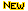Top Free Python Books - 100% Free or Open Source!
• Title: Computability and Randomness
• Author(s) Andre Nies
• Publisher: Oxford University Press (May 16, 2012); eBook (Final Draft of 2008 Edition)
• Paperback: 456 pages
• eBook: PDF (447 pages)
• Language: English
• ISBN-10: 0199652600
• ISBN-13: 978-0199652600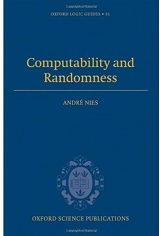Book Description

The interplay between computability and randomness has been an active area of research in recent years, reflected by ample funding in the USA, numerous workshops, and publications on the subject. The complexity and the randomness aspect of a set of natural numbers are closely related.

Traditionally, computability theory is concerned with the complexity aspect. However, computability theoretic tools can also be used to introduce mathematical counterparts for the intuitive notion of randomness of a set. Recent research shows that, conversely, concepts and methods originating from randomness enrich computability theory.

The book covers topics such as lowness and highness properties, Kolmogorov complexity, betting strategies and higher computability.

Both the basics and recent research results are desribed, providing a very readable introduction to the exciting interface of computability and randomness for graduates and researchers in computability theory, theoretical computer science, and measure theory.

The book is intended for graduate students and researchers in mathematical logic, foundations of mathematics, and theoretical computer science. It includes a detailed treatment of Turing's theory of computability and unsolvability as subsequently developed by Kleene, Post, Friedberg, and many other researchers up to the present.

• N/A

Reviews, Ratings, and Recommendations: Related Book Categories: Read and Download Links:Similar Books:
•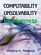Computability, Unsolvability, Randomness (Stephen G. Simpson)

The author exposits Turing's 1936 theory of computability and unsolvability, as subsequently developed by Kleene and Post. This theory is of the essence in theoretical computer science and in the study of unsolvable mathematical problems.

•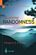Exploring Randomness (Gregory J. Chaitin)

This book presents the technical core of the theory of program-size complexity. LISP is used to present the key algorithms and to enable computer users to interact with the authors proofs and discover for themselves how they work.

•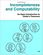Incompleteness and Computability: Gödel's Theorems

This book is an introduction to metamathematics and Gödel's Theorems. It covers recursive function theory, arithmetization of syntax, the first and second incompleteness theorem, models of arithmetic, second-order logic, and the lambda calculus.

•Introduction to Probability, Statistics, and Random Processes

This book introduces students to probability, statistics, and stochastic processes. It provides a clear and intuitive approach to these topics while maintaining mathematical accuracy. The book contains a large number of solved exercises.

•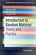Introduction to Random Matrices: Theory and Practice

This is a book for absolute beginners. The aim is to provide a truly accessible introductory account of Random Matrix theory. Most chapters are accompanied by MATLAB codes to guide readers through the numerical check of most analytical results.

•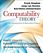Computability Theory: Introduction to Recursion Theory

Computability Theory originated with the seminal work of Gödel, Church, Turing, Kleene and Post in the 1930s. These texts provide concise, comprehensive, and authoritative introduction to contemporary computability theory, techniques, and results.

•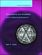Computability and Complexity: From a Programming Perspective

This book is an introduction to the basic concepts of computability, complex, and the theory of programming languages. Its goal is to build a bridge between computability and complexity theory and other areas of computer science, especially programming.

•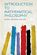Introduction to Mathematical Philosophy (Bertrand Russell)

Requiring neither prior knowledge of mathematics nor aptitude for mathematical symbolism, the book serves as essential reading for anyone interested in the intersection of mathematics and logic and in the development of analytic philosophy.

•What is Mathematics: Godel's Theorem and Around (K. Podnieks)

This accessible book gives a new, detailed and elementary explanation of the Godel incompleteness theorems and presents the Chaitin results and their relation to the da Costa-Doria results, which are given in full, but with no technicalities.

Book Categories
 :All CategoriesTop BooksRecent BooksMiscellaneous BooksComputer LanguagesComputer ScienceData Science/DatabasesElectrical EngineeringJava and Java EE (J2EE)Linux and UnixMathematicsMicrosoft and .NETMobile ComputingNetworking and CommunicationsSoftware EngineeringSpecial TopicsWeb Programming
Other Categories